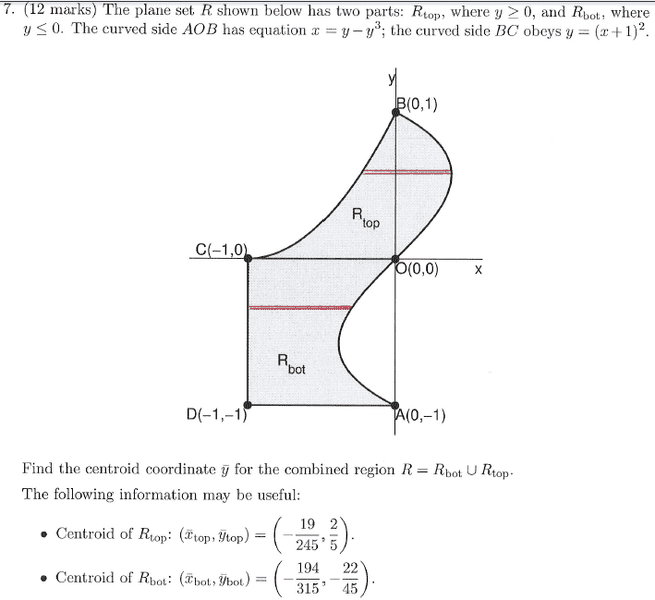# Finding the combined centroid of two regions given the centroids of both regions

## Homework Statement## The Attempt at a Solution

I tried to do this problem by taking the average of the y-bar centroid values but that gave me the wrong answer. I am only interesting in knowing why this method is incorrect.

Thanks!

## Answers and Replies

SteamKing
Staff Emeritus
Science Advisor
Homework Helper
What is the definition of centroid? Your method would be valid if the areas of the two regions were equal.

HallsofIvy
Science Advisor
Homework Helper
It can be shown that the centroid of the union of two (non-overlapping) regions is the weighted average of the two centroids, weighted by the areas of the regions. That is, if the two regions have centroid $(x_1, y_1)$ and $(x_2, y_2)$ and have areas $A_1$ and $A_2$, respectively, then the centroid of the combined regions is at
$$\left(\frac{A_1x_1+ A_2x_2}{A_1+ A_2}, \frac{A_1y_1+ A_2y_2}{A_1+ A_2}\right)$$

haruspex
Science Advisor
Homework Helper
Gold Member
2020 Award

I tried to do this problem by taking the average of the y-bar centroid values but that gave me the wrong answer.
As your grandmother should have taught you, don't take an average of averages. If an elephant and a flea get on opposite ends of a symmetric seesaw, will they balance? So where's their combined centroid?